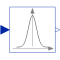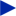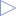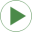# UniformDensity

Calculates the density of a uniform distribution# Information

This information is part of the Modelica Standard Library maintained by the Modelica Association.

This block determines the probability density y of a uniform distribution for the given input signal u (for details of this density function see Math.Distributions.Uniform.density).

This block is demonstrated in the example Examples.NoiseExamples.Densities .

# Parameters (2)

u_min Value: Type: Real Description: Lower limit of u Value: Type: Real Description: Upper limit of u

# Connectors (2)

u yType: RealInput Description: Real input signalType: RealOutput Description: Density of the input signal according to the uniform probability density function

# Used in Examples (1)Densities Modelica.Blocks.Examples.NoiseExamples Demonstrates how to compute distribution densities (= Probability Density Function)